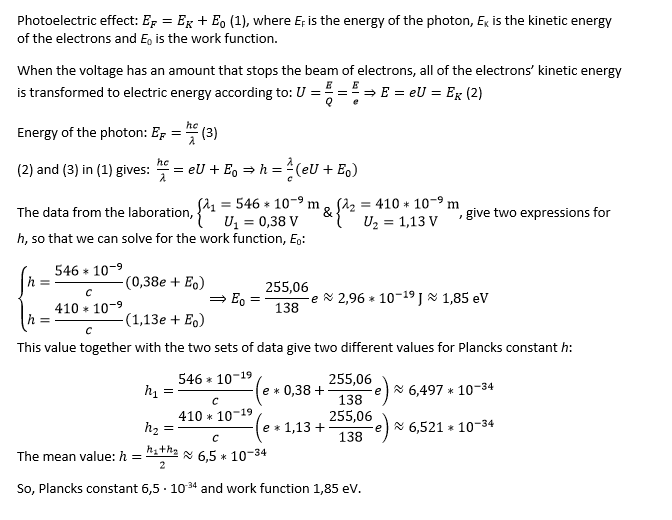# Photoelectric Effect - Laboration

## Homework Statement

"During a laboratory experiment with photoelectric effect, a metal plate is irradiated with light. The voltage that completely stops the beam of electrons is then measured.

When the wavelength is 546 nm, the voltage is 0,38 V. When the wavelength is 410 nm, the voltage is 1,13 V.

Calculate an experimental value for Planck's constant and the work function of the metal plate."

## Homework Equations

$E_{F} = E_{K} + E_{0} \\ E_{F} = \frac{hc}{\lambda} \\ U = \frac{E}{Q} \\$

## The Attempt at a SolutionWhat I am wondering is, have I solved the problem correctly? I know many would address the problem by making a graph with the kinetic energy on the y-axis and the frequency on the x-axis... Thus I am not sure if my way works, however I believe it should...
So: Is my solution correct?

•